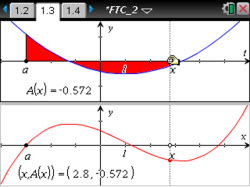Activities

••• Subject Area

• Math: Calculus: Fundamental Theorem

• Author9-12

45 Minutes

• Device
•TI-Nspire™ CX/CX II
•TI-Nspire™ CX CAS/CX II CAS
• TI-Nspire™ Navigator™
• TI-Nspire™
• TI-Nspire™ CAS
• Software

TI-Nspire™
TI-Nspire™ CAS

3.0

• Report an Issue

The Second Fundamental Theorem of CalculusActivity Overview

Students make visual connections between a function and its definite integral.

Objectives

• Students will be able to identify the graphical connections between a function and its accumulation function.
• For a given function, students will recognize the accumulation function as an antiderivative of the original function.
• Students will be able to apply and explain the second Fundamental Theorem of Calculus.
• Construct viable arguments and critique the reasoning of others. (CCSS Mathematical Practice)
• Look for and make use of structure. (CCSS Mathematical Practice)

Vocabulary

• accumulation function
• definite integral
• antiderivative